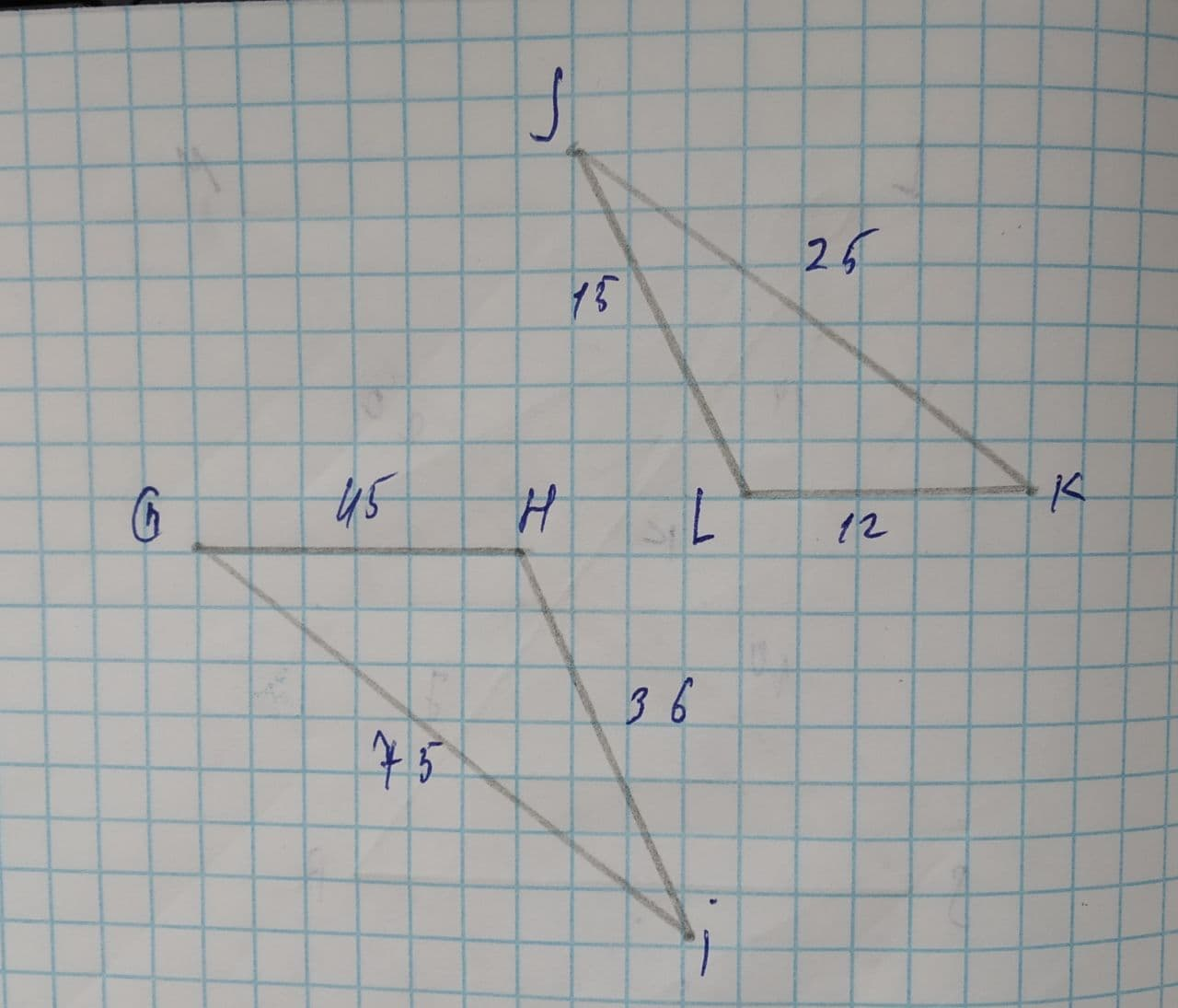Determine whether the two triangles are similar \triangle GHI\sim \triangle. If they are, complete the similarity statement.Lennie Carroll 2021-08-02 Answered
Determine whether the two triangles are similar. If they are, complete the similarity statement.
Select all that apply.
$$\displaystyle\triangle{G}{H}{I}\sim\triangle$$ ? byThe triangles are not similar.
$$\displaystyle\triangle{G}{H}{I}\sim\triangle{K}{L}{J}$$
$$\displaystyle\triangle{G}{H}{I}\sim\triangle{J}{L}{K}$$
By SAS similarity
By SSS similarity
By AA similarity

• Questions are typically answered in as fast as 30 minutes

Solve your problem for the price of one coffee

• Math expert for every subject
• Pay only if we can solve itClara Reese
Step 1
The figure of the triangles are given byStep 2
From the figure we can write as
$$\displaystyle{\frac{{{G}{H}}}{{{J}{L}}}}={\frac{{{45}}}{{{15}}}}={3}$$...(1)
$$\displaystyle{\frac{{{H}{I}}}{{{L}{K}}}}={\frac{{{36}}}{{{12}}}}={3}$$...(2)
$$\displaystyle{\frac{{{G}{I}}}{{{J}{K}}}}={\frac{{{75}}}{{{25}}}}={3}$$...(3)
From equations (1), (2) and (3) we get,
$$\displaystyle{\frac{{{G}{H}}}{{{J}{L}}}}={\frac{{{H}{I}}}{{{L}{K}}}}={\frac{{{G}{I}}}{{{J}{K}}}}$$...(3)
Relation (3) states that all the three corresponding sides of the triangles are proportional.
So, the triangles $$\displaystyle\triangle{G}{H}{I}\sim\triangle{J}{L}{K}$$ by SSS similarity.